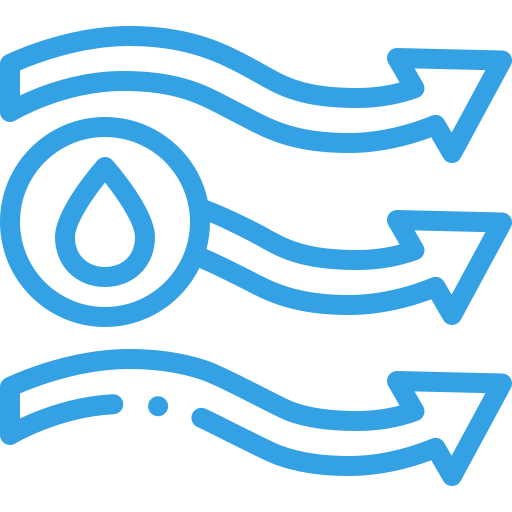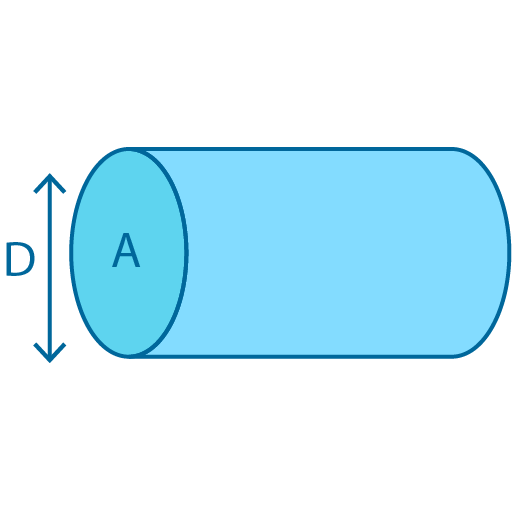•Sign In
• Blog
• Write for UsUh Oh! It seems you’re using an Ad blocker!

We always struggled to serve you with the best online calculations, thus, there's a humble request to either disable the AD blocker or go with premium plans to use the AD-Free version for calculators.

Or# Flow Rate Calculator

Select method, shape, and write down the required parameters in designated fields to calculate the volumetric flow rate of fluids through this calculator.

Solve Via

Select ShapeVolume

Time

Diameter(d)

Flow Velocity

Liquid Density

Filled

Height

Width

Cross-sectional area(A)

Top Width

Bottom Width

Pressure Start

Pressure End

Pipe Length

Dynamic Viscosity

Table of Content

 1 What is priceeight Class? 2 priceeight Class Chart: 3 How to Calculate priceeight Density (Step by Step): 4 Factors that Determine priceeight Classification: 5 What is the purpose of priceeight Class? 6 Are mentioned priceeight Classes verified by the officials? 7 Are priceeight Classes of UPS and FedEx same?

Get the Widget!

Add this calculator to your site and lets users to perform easy calculations.

Feedback

How easy was it to use our calculator? Did you face any problem, tell us!

Use this flow rate calculator to calculate the average volumetric flow rate of fluids regarding flow velocity, pressure difference, time & volume. With this pipe flow rate calculator, you will not only calculate the flow rate of a garden hose or showerhead but also find out how much blood your heart pumps every minute. What a function! Isn’t it?

If you want to determine this particular term, then the read below is specially arranged for you. So let’s get through it together!

## Flow Rate:

“The volume of fluid passing via a given cross-sectional area per unit is called flow rate of the fluid”.

The term cross-sectional area is often used to describe the area through which liquid is flowing.

### Formula:

Get going for determining the flow rate of the fluid by subjecting to the following equation:

Q=V/T

Or

Flow rate= volume/time

### Flow Velocity:

The vector field that provides the velocity of fluids at a specific time and position is known as flow velocity in the fluid.

#### Formula:

The following is the equation to determine the flow velocity of any fluid:

Q=AV

Where,

Q = represents the liquid flow rate measured as m3/s or L/s

A = represents the area of the pipe in m2

v = represents the velocity of the liquid in m/s

### Mass Flow Rate:

The mass flow rate is the mass of fluids that pass through per unit of time.

#### Formula:

mass= dm/dt

Or

mass=ρVA

Where

ρ = displays the density of the fluid

V = represents the velocity of the liquid

A = represents area of cross-section

### Pressure Difference:

The pressure difference of fluids is the force that exists per unit and is measured in pounds per square inch (PSI). It is also equivalent to the unit that is subtracted from a greater force per unit.
Here you can calculate the flow rate of pressure difference by using this best water flow calculator

#### Steps to calculate pressure difference of fluid:

• Firstly, find out the square of the volumetric flow rate.
• Calculate the square of the flow factor.
• Divide the square of volumetric flow rate by the square of flow factor.
• Multiply the resultant with the specific gravity of the fluid to obtain the differential pressure.

### Volume & Time:

The volumetric flow rate of fluids is the volume that passes per unit of time.

Generally, water flow through pipe calculator is a convenient and straightforward way to calculate the volume of fluid passing through in a given time.

### How To Calculate Flow Rate?

Example # 01

Suppose water is flowing from a circular pipe with a radius of 0.0900 m. Furthermore, its velocity is 4.40 m/s. So, calculate the flow rate of water that flows from the pipe in liters per second (L/s)?

Solution:

First of all, find the area of the circular pipe for water flow calculation.

A= 𝜋r^2

A= 𝜋(0.0900m)^2

A= 3.14*0.0081m^2

A= 0.025434m^2

So the area of the pipe is 0.025434m^2

Now we can find the flow rate

Q=AV

Q= (0.025434m^2)(4.40 m/s)

Q= 0.1119096m^3/s

Furthermore, we can convert it into liters per second: 1m^3/s= 1000L/s

Q= 0.1119096m^3/s * 1000L/s / 1m^3/s

Q= 111.9096L/s

Example # 02

A liquid with a density of 1000kg/m^3 flows through a circular pipe whose diameter is 7cm. The velocity of the liquid is 10.5m/s. Find the mass flow rate of the liquid?

Solution:

Density= 1000kg/m^3

Diameter= 7cmVelocity= 10.5m/s

Radius of the pipe=r= d/2 =7/2 = 3.5m

Area of the pipe=A= 𝜋r^2= 3.14*(3.5m)^2

=3.14*12.2

=38.465m

Volumetric flow rate=Q= VA

=(10.5m/s)*(38.465m)=403.8825m^3/s

Mass flow rate =m= Q*ρ

=(403.8825m^3/s)*(1000kg/m^3)

=403,882.5kg/s

### How Does The Water Flow Rates Calculator Work?

Let’s see this free flow rate calculator find out the parameters of flow rate through pipe given pressure. Let’s move on and

have a look at the working of this calculator.

Input:

• First of all, hit the drop-down menu and select the parameter in which you want either to calculate such as flow velocity, pressure difference, time & volume.
• After that, enter the values of different parameters according to your selection.
• Enter the units
• Make a tap on the calculate button

Output:

The free volume flow rate calculator does the following calculations

• Determines the volumetric flow rate and the mass flow rate
• Also this calculator helps you to display the relative units conversion against the output

## FAQs:

### Are the flow rate and velocity of the fluids the same?

No, both are different phenomena. Flow rate is the measure of fluid in terms of volume per unit. While velocity tells how fast fluids are moving in distance per unit of time.

### What factors affect the flow rate in a pipe?

There are some factors that affect fluid flow in pipes including viscosity, density, and velocity of the fluid. Changes in the fluid temperature will change the viscosity & density of the fluid.

### Why is pressure the same in all directions in a fluid?

All fluids exert pressure like the air inside a tire. The particles of fluids are constantly moving in all directions randomly. As the particles move, they keep striking into each other and into anything else in their path. These collisions cause pressure, and therefore, the pressure is exerted equally in all directions

### Write two factors that affect the pressure in a fluid?

Factors affecting pressure in a liquid

• The density of the liquid (ρ)
• Depth of liquid (h)
• Acceleration due to gravity (g)

### Does pipe length affect pressure?

No, as friction pushes against the flow that may result in pressure loss as the pipe length increases. As flow velocity increases, the pressure loss increases, and efficiency decreases.

## Conclusion:

Flow rate is the fluid volume used for an appropriate flowmeter to ensure fluid control in different industries. Flowmeter technologies such as differential pressure, turbine, ultrasonic, magnetic, thermal, and vortex all measured flow rate as a function of fluid velocity. Flowmeter users who know what flow rate they need to measure can use a flow rate calculator to determine the fluids.

## References:

From the source of Wikipedia: Volumetric flow rate, Mass flow rate

From the source of khan academy:  volume flow rate, Incompressibility of liquids